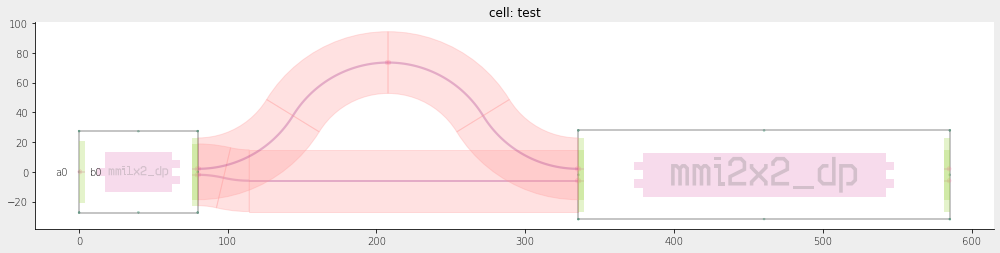Home Forums Nazca Create trial cells when using minimizer algorithm?

Viewing 6 posts - 1 through 6 (of 6 total)
• Author
Posts
• #6427

Hello,
I have to layout asymmetric MZIs with an exact path length difference. The overall path lengths should be no longer than necessary. Normally I solve the trigonometry problem on paper, then code it as a Python function.
Since there’s typically not a closed-form solution I use scipy.minimize() to find function parameters that get the right path length difference and also make the waveguides connect up correctly.

This works, but I wonder if there’s a faster way to code it up than having to solve all this math for each geometry I try. Can I create a new cell with each iteration within scipy.minimize(), never place the cell in the layout, and use the a0/b0 ports’ .xya() data to make sure that my waveguides will join up ok? Do I need to do some cleanup after each iteration to avoid running out of memory or filling the netlist with cells I will never use? I see there’s a cell.close() method but I’m not sure if that does what I want, which is to erase the cell from memory & netlist as if it was never there.

By the way it also doesn’t seem appropriate to use interconnect functions for this application since I don’t think there is a way to get them to return path lengths or minimum curvature in an interconnect.

Thank you!
Jon

#6431

I set up a problem using trial cells with the purpose of finding path length & exact output port location. The approach does work. But I still haven’t figured out how to remove a cell from the netlist.

I get very many warnings like this:
WARNING: netlist.py: ND-342: Duplicate cell name in nd.Cell(name=’trial bot’) renamed to ‘trial bot_1506’.

I realized that cell.close() is automatically called when I use the “with nd.Cell(…) as cell:” formation, so that is not the method I’m looking for.

Standard python “del cell” does not seem to work.

The unused trial cells don’t show up in my GDS hierarchy, so they aren’t increasing my GDS file size. The only issue remains that if I adopt this practice of making trial cells and build bigger layouts with it, I’ll run out of memory or have slower compiles.

Thanks, Jon

#6444

Dear Jon,

The below MZI example in demofab may contain what you are looking for.

A root-solver optimizes the MZI for a specific target geometrical path length difference. The MZI method behaves either like a function to optimize for a path length difference in the root-solver, or it returns a Nazca Cell. In the former case it deletes all cells generated in the call; Particularly related to your question, I create sets S1 and S2 from dict nd.cfg.cellnames just before and just after a cell iteration. This dict is leading in tracking cell warnings etc., and popping the new cells S2 – S1 will make Nazca forget about them.

The example here uses the “trace” module for measuring geometrical interconnect length, but this concept also can be exploited in a more fancy way utilizing the pathfinder module and compact models, which is something that would need dedicated tutorials.

As for your radius question, interconnects do have a build-in minimum radius check option, which is based on the xsection.minimum_radius attribute of the xs you assign to the interconnect.

``````from functools import partial
from scipy.optimize import fsolve
import nazca as nd
import nazca.demofab as demo

def cell_to_solve(offset=10, target_diff=20, cell=False):
"""Create a temporary MZI cell to return the arm length difference or return the Cell.

Free parameter here is the sbend offset.

Args:
cell (bool): False: return distance from target; True: Return MZI cell.

Returns:
Cell | float:
"""
if not cell:
S1 = set(nd.cfg.cellnames.keys())
with nd.Cell('test') as C:
m1 = demo.mmi1x2_dp().put(0)
nd.trace.trace_start()
demo.deep.sbend(offset=offset).put(m1.pin['b0'])
demo.deep.sbend(offset=-offset).put()
nd.trace.trace_stop()
L1 = nd.trace.trace_length()
m2 = demo.mmi2x2_dp().put()
nd.trace.trace_start()
demo.deep.sbend_p2p(pin1=m1.pin['b1'], pin2=m2.pin['a1']).put()
nd.trace.trace_stop()
L2 = nd.trace.trace_length()
diff = L1 - L2
if cell:
return C
else:
S2 = set(nd.cfg.cellnames.keys())
for dS in S2 - S1:
nd.cfg.cellnames.pop(dS)
return diff - target_diff

def find_MZI_solution(target_diff, initial_offset):
"""Find an optimized offset to reach the target-diff in the MZI using a standard root solver.

Args:
target_diff (float): target armlength difference in MZI
inititial_offset (float): starting offset to find a solution
"""
func = partial(cell_to_solve, target_diff=target_diff)
root = fsolve(func, [initial_offset])
return func(offset=root, cell=True)

MZI = find_MZI_solution(target_diff=50, initial_offset=70)
nd.export_png(MZI)``````

Ronald

#6445

The resulting MZI as reference, for offset = 71.4428629.Ronald

#6446

A decorator can clean up the code a bit like this:

``````def trial_cell(func):
def wrapper(*args, **kwargs):
cell = kwargs.pop('cell', False)
if not cell:
S1 = set(nd.cfg.cellnames.keys())
out = func(*args, cell=cell, **kwargs)
if not cell:
S2 = set(nd.cfg.cellnames.keys())
for s in S2 - S1:
nd.cfg.cellnames.pop(s)
return out
return wrapper

@trial_cell
def cell_to_solve(offset=10, target_diff=20, cell=False):
with nd.Cell('test') as C:
m1 = demo.mmi1x2_dp().put(0)
nd.trace.trace_start()
demo.deep.sbend(offset=offset).put(m1.pin['b0'])
demo.deep.sbend(offset=-offset).put()
nd.trace.trace_stop()
L1 = nd.trace.trace_length()
m2 = demo.mmi2x2_dp().put()
nd.trace.trace_start()
demo.deep.sbend_p2p(pin1=m1.pin['b1'], pin2=m2.pin['a1']).put()
nd.trace.trace_stop()
L2 = nd.trace.trace_length()
diff = L1 - L2
if cell:
return C
else:
return diff - target_diff``````

Ronald

#6447

Ronald,
Thank you so much! I tried the examples you gave and I understand them. I was able to partially implement it in my code, though not in every instance. I think it’s pretty hard to diagnose what is going wrong for me due to complicated code structure. It seems like good style for Nazca is to have one cell per function, so I’ll stick with this in future designs.

Next time I code a structure using a root finder approach I’ll follow the style of your example.

Best,
Jon

Viewing 6 posts - 1 through 6 (of 6 total)
• You must be logged in to reply to this topic.